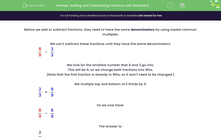# Adding and Subtracting Fractions with Related Denominators

In this worksheet, students add and subtract fractions, where the denominator of one fraction is a multiple of the other.Key stage:  KS 2

Curriculum topic:   Number: Fractions, Decimals and Percentages

Popular topics:   Fractions worksheets

Difficulty level:#### Worksheet Overview

Before we add or subtract fractions, they need to have the same denominators by using lowest common multiples.

We can't subtract these fractions until they have the same denominators:

 8 - 2 9 3

We look for the smallest number that 9 and 3 go into.

This will be 9, so we change both fractions into 9ths.

(Note that the first fraction is already in 9ths, so it won't need to be changed.)

We multiply top and bottom of 2 thirds by 3:

 2 = 6 3 9

So we now have:

 8 - 6 9 9

 2 9

Example

Work out the following, remembering to give your answer in its lowest terms.

 7 + 4 15 30

The lowest common multiple of 15 and 30 is 30, because 2 × 15 = 30.

We multiply top and bottom of 7 fifteenths by 2:

 7 = 14 15 30

So we now have:

 14 + 4 30 30

This gives:

 18 30

But this must be reduced by dividing top and bottom by 2.

 9 15

which we write as   9/15.

### What is EdPlace?

We're your National Curriculum aligned online education content provider helping each child succeed in English, maths and science from year 1 to GCSE. With an EdPlace account you’ll be able to track and measure progress, helping each child achieve their best. We build confidence and attainment by personalising each child’s learning at a level that suits them.

Get started